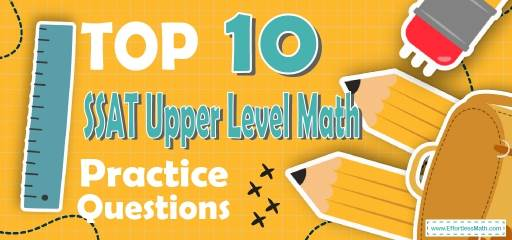# Top 10 SSAT Upper Level Math Practice QuestionsPreparing for the SSAT Upper-Level Math test? The best way to prepare for your SSAT Upper-Level Math test is to work through as many SSAT Upper-Level Math practice questions as possible. Here are the top 10 SSAT Upper-Level Math practice questions to help you review the most important SSAT Upper-Level Math concepts. These SSAT Upper-Level Math practice questions are designed to cover mathematics concepts and topics that are found on the actual test. The questions have been fully updated to reflect the latest 2022 SSAT Upper-Level guidelines. Answers and full explanations are provided at the end of the post.

Start your SSAT Upper-Level Math test prep journey right now with these samples SSAT Upper-Level Math questions.

## SSAT Upper Level Math Practice Questions

1- A school wants to give each of its $$22$$ top students a football ball. If the balls are in boxes of four, how many boxes of balls do they need to purchase?

A.2

B. 4

C. 6

D. 8

E. 22

2- A shaft rotates $$240$$ times in $$12$$ seconds. How many times does it rotate in $$20$$ seconds?

A. 450

B. 400

C. 300

D. 250

E.200

3- What is the value of $$x$$ in the following figure?

A. 150

B. 145

C. 135

D. 125

E. 115

4- $$14.125÷0.005?$$

A. 2.825

B. 28.250

C. 282.50

D. 2825

E.28025

5- How many tiles of $$5$$ cm$$^2$$ are needed to cover a floor of dimension $$5$$ cm by$$20$$ cm?

A.5

B. 10

C. 15

D. 20

E. 25

6- If $$\frac{30}{A}+1=7$$, then $$30+A= ?$$

A. 2

B. 7

C. 35

D. 40

E. 50

7- What is the value of the sum of the tens and thousandths in number $$3,617.89652$$?

A. 16

B. 11

C. 8

D. 14

E. 6

8- Jack earns $$\720$$ for his first $$45$$ hours of work in a week and is then paid $$1.5$$ times his regular hourly rate for any additional hours. This week, Jack needs $$\936$$ to pay his rent, bills, and other expenses. How many hours must he work to make enough money this week?

A. 50

B. 54

C. 60

D. 63

E. 64

9- If $$50\%$$ of a number is $$85$$, then what is the $$20\%$$ of that number?

A. 34

B. 40

C. 50

D. 60

E. 85

10-

Which of the following statements is correct, according to the graph below?

A. Number of books sold in April was twice the number of books sold in July.

B. Number of books sold in July was less than half the number of books sold in May.

C. Number of books sold in June was half the number of books sold in April.

D. Number of books sold in July was equal to the number of books sold in April plus the number of books sold in June.

E. More books were sold in April than in July.

## Best SSAT Upper Level Math PrepResource for 2022

1- C
Several packs equal to $$\frac{22}{4}≅5.5$$, therefore, the school must purchase $$6$$ packs.

2- B
First create a proportion: $$\frac{240}{12}=\frac{x}{20}$$, Number of rotates in $$20$$ second equals to: $$\frac{240×20}{12}=400$$

3- A
$$x=30+120=150$$

4- D
$$14.125÷0.005=\frac{\frac{14,125}{1,000}}{\frac{5}{1,000}}=\frac{14,125}{5}=2,825$$

5- D
The area of the floor is $$5$$ cm $$×20$$ cm $$=100$$ cm$$^2$$, the number of tiles needed $$= 100÷5=20$$

6- C
$$\frac{30}{A}+1=7→\frac{30}{A}=7-1=6 →30=6A→A=\frac{30}{6}=5, 30+A=30+5=35$$

7- D
The digit in tens place is $$8$$. The digit in the thousandth place is $$6$$. Therefore; $$8+6=14$$

8- B
The amount of money that Jack earns for one hour: $$\frac{720}{45}=16$$
The number of additional hours that he needs to work in order to make enough money is: $$\frac{936-720}{1.5×16}=9$$, number of total hours Jack must work is: $$45+9=54$$

9- A
First, find the number. Let $$x$$ be the number. Write the equation and solve for $$x$$.

10- C
A. Number of books sold in April is: $$380$$
Number of books sold in July is: $$760→ \frac{380}{760}=\frac{38}{76}=\frac{1}{2}$$
B. Number of books sold in July is: (760)
Half the number of books sold in May is: $$\frac{1140}{2}=570→760>570$$
C. Number of books sold in June is: (190)
Half the number of books sold in April is: $$\frac{380}{2}=190→190=190$$
D. $$380+190=570<760$$
E. $$380<760$$

Looking for the best resource to help you succeed on the SSAT Upper-Level Math test?

## The Best Books to Ace the SSAT Upper Level Math Test

### What people say about "Top 10 SSAT Upper Level Math Practice Questions - Effortless Math: We Help Students Learn to LOVE Mathematics"?

No one replied yet.

X
52% OFF

Limited time only!

Save Over 52%

SAVE $40 It was$76.99 now it is \$36.99